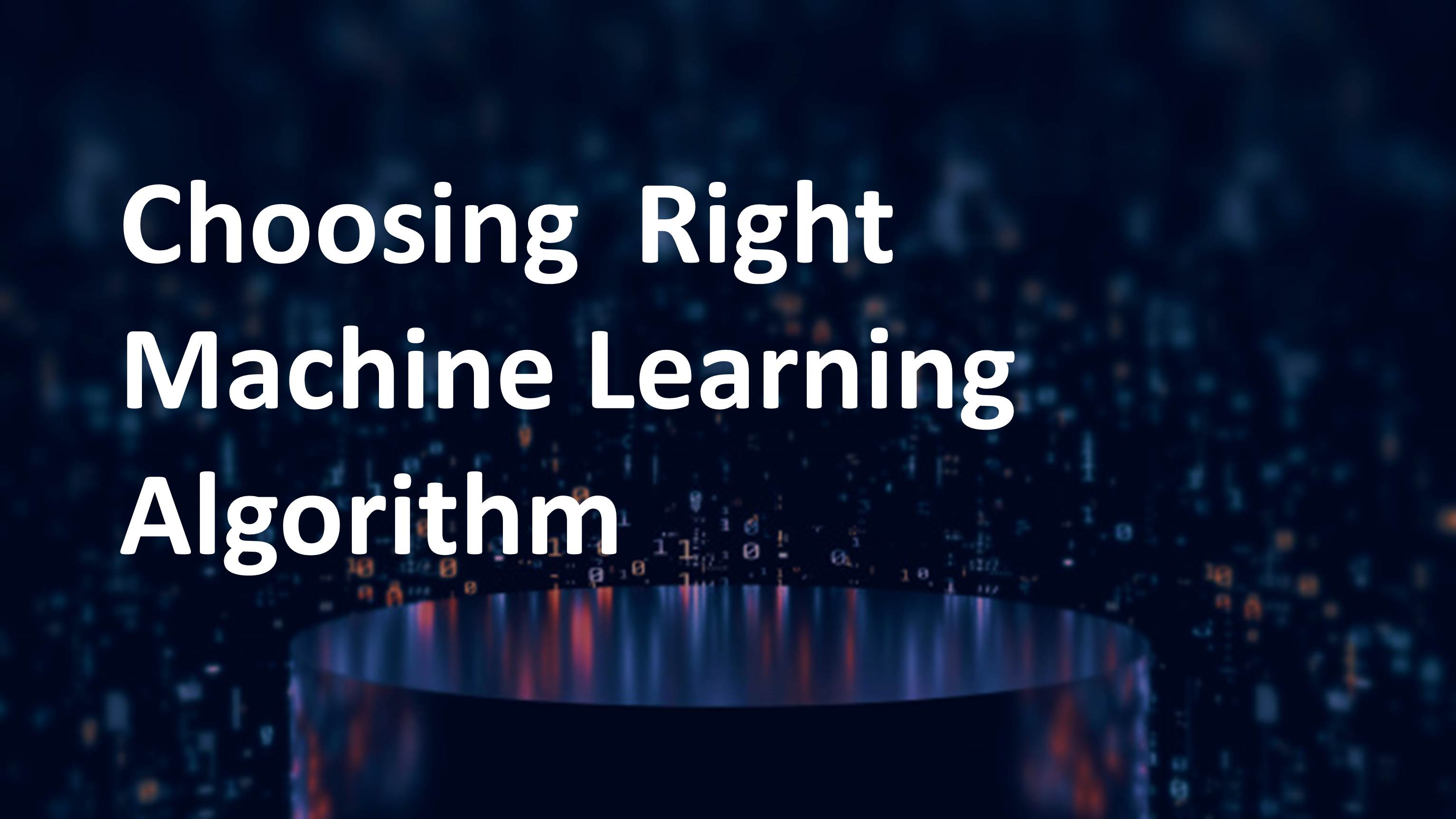/  Technology   /  Confused about choosing the right Machine Learning algorithm?## Confused about choosing the right Machine Learning algorithm?

Well, there is no straightforward answer to this question. It depends on many factors like the problem statement and the kind of output you want, type and size of the data, the available computational time, number of features, and observations in the data and many more.

Here I am listing few important things for choosing an algorithm

1. Categorize the problem

Categorize by the input: If input data is a labeled data, it’s a supervised learning problem. If it’s unlabeled data it’s an unsupervised learning problem. If the solution implies to optimize an objective function by interacting with an environment, it’s a reinforcement learning problem.
Categorize by output: If the output of the model is a number, it’s a regression problem. If the output of the model is a class, it’s a classification problem. If the output of the model is a set of input groups, it’s a clustering problem.

1. Size of the training data

Data is the main raw material in the whole analysis process. Understanding the data plays a key role in selecting the right algorithm for the right problem. Few algorithms will best suit for categorical data while others suit best for numerical data input. It is also recommended to gather a good amount of data to get better predictions. If the training data is smaller or if the dataset has a fewer number of observations and a higher number of features like genetics or textual data, choose algorithms with high bias/low variance like Linear regression, Naïve Bayes, or Linear SVM.

If the training data is sufficiently large and the number of observations is higher as compared to the number of features, one can go for low bias/high variance algorithms like KNN, Decision trees, or kernel SVM.

3. Performance and/or Interpretability of the output

Performance metrics like Precision, Recall, Loss need to be analyzed. Do not just stick onto one or two metrics and decide the model. There are two important tasks which are realize data with descriptive statistics and realize data with visualization and plots.

4. Speed or Training time and space optimization

Few algorithms take more time to train on large training data. Algorithms like Naïve Bayes and Linear and Logistic regression are easy to implement and fast to run. Algorithms like SVM, which involve tuning of parameters, Neural networks with high convergence time, and random forests, need a lot of time to train the data. For a model to be trained we should not use too many resources.

5. Linearity

Many algorithms work on the assumption that classes can be separated by a straight line. For example, logistic regression and support vector machines. If the data is linear, then Linear regression and support vector machines algorithms perform quite good.

Sometimes we require other algorithms which can handle high dimensional and complex data structures. Examples include kernel SVM, random forest, neural nets.

Try to fit a linear line or run a logistic regression or SVM and check for residual errors. A higher error means the data is not linear and would need complex algorithms to fit.

6. Number of features

Some dataset might have large number of features which can bog down some learning algorithms. This causes training time unfeasibly long. PCA and feature selection methods helps to decrease dimensionality and select important features.

1. Optimize hyperparameters

Grid search, random search, and Bayesian optimization are the three options for optimizing hyperparameters

The main points to consider when trying to solve a new problem are:

• Define the problem. What is the objective of the problem?
• Explore the data and familiarize yourself with the data.
• Start with basic models to build a baseline model and then try more complicated methods.